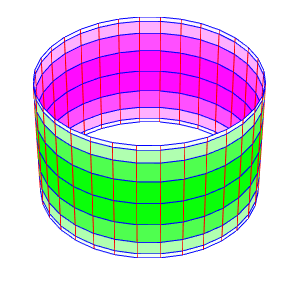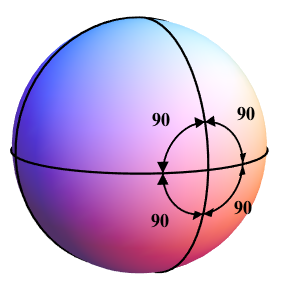# Diaspora

## Chapter 2: Truth Mining

### Flattening a torus without squashing it

Torus embedded in 3 dimensions Torus embedded in 4 dimensions (4th coordinate shown by shading) The surface of a torus embedded in 3 dimensions is intrinsically curved (below left). At first glance, it might seem that the only way to make the surface flat would involve squashing the meridians (red circles) into straight lines, to allow all the lines of latitude (blue circles) to have equal radius. But that would change the topology of the surface; it would simply become a cylinder. However, by rotating each meridian into a 4th spatial dimension, the circles of latitude can be made equal without squashing the meridians. The result looks like a cylinder when seen in 3 dimensions (below right), but the different shading on the inner and outer surfaces indicate that they’re not actually touching, but are separated in the 4th dimension. More detail.### Why a sphere can’t be flattened

A sphere divided into 8 triangles, with 360 degrees around each vertex The same sphere deformed so that each triangle is Euclidean, with angles summing to 180 degrees To see why a sphere can’t be flattened by embedding it in any number of higher dimensions, picture it divided into eight triangles (below left). There are 6 points where 4 triangles meet, and if the surface is flat the angles around each point must sum to 360 degrees, making a total of T = 6 × 360 = 2160 degrees. But it must also be true that the angles of each triangle sum to 180 degrees (below right), and since there are 8 triangles the total must be T = 8 × 180 = 1440 degrees. These two conditions are both necessary for the surface to be flat — and they can’t both be true. More detail.Diaspora / Chapter 2: Truth Mining / created Saturday, 25 October 1997 / revised Monday, 8 December 1997